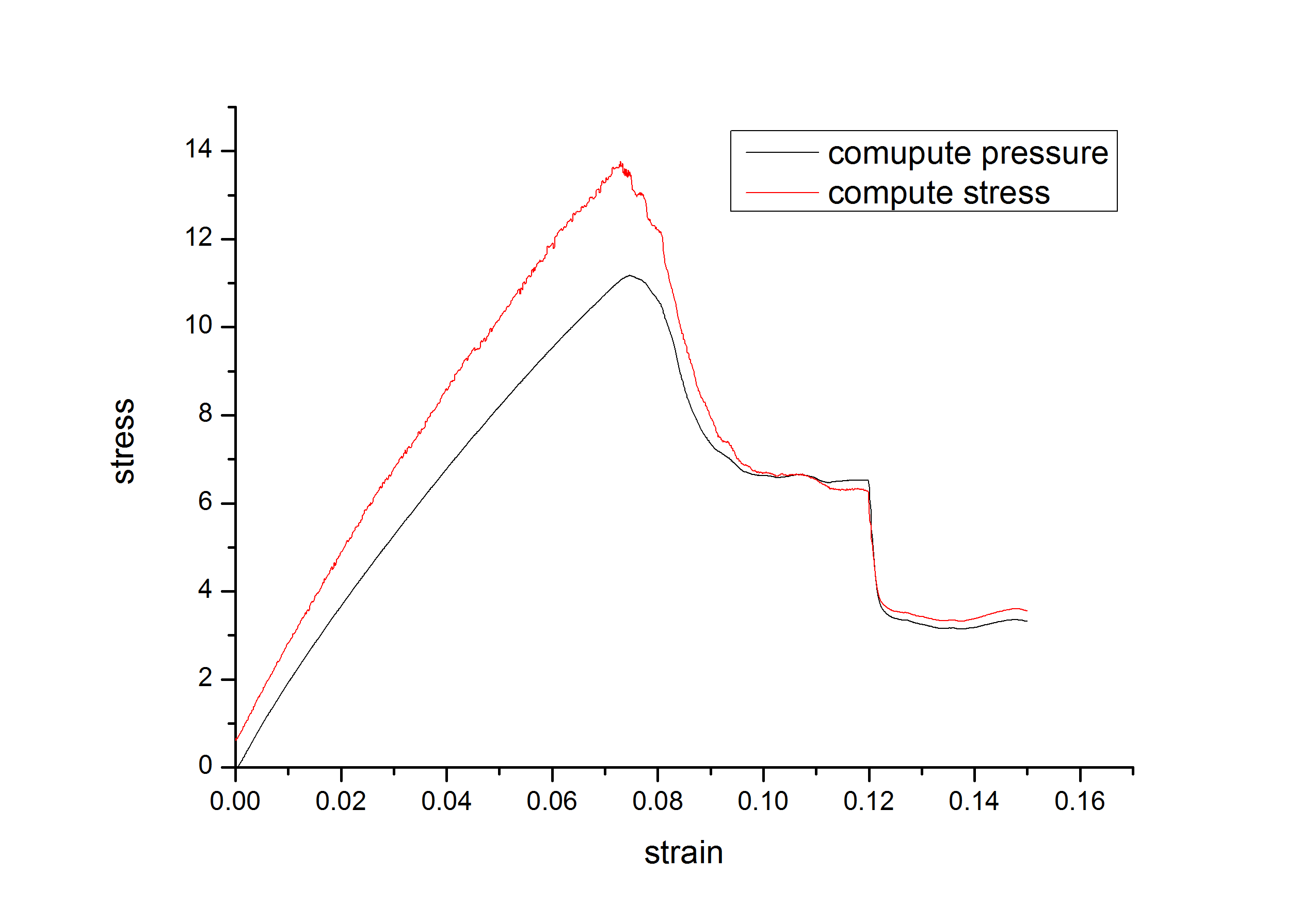# The difference between compute pressure and compute stress/atom

Hi,

Recently, I adopted the compute pressure and compute stress/atom to calculate normal stress under uniaxial tensile as shown in the attached Figure, here the tensile direction is with the periodic boundary, and the other two direction are the free surface, I found that the stresses are not equal at the beginning, although they are in agreement at the end of progress, so what is the reason, they are all based on the virial theory. I also test under all three directions are the periodic boundaries, this time they are nearly coincident.

Best wishes!Hi,

Recently, I adopted the compute pressure and compute stress/atom to
calculate normal stress under uniaxial tensile as shown in the attached
Figure, here the tensile direction is with the periodic boundary, and the
other two direction are the free surface, I found that the stresses are not
equal at the beginning, although they are in agreement at the end of
progress, so what is the reason, they are all based on the virial theory. I
also test under all three directions are the periodic boundaries, this time
they are nearly coincident.

first rule about ​figures in science: *always* include units!

since you don't explain with sufficient detail what you are computing for
the figure, there is little to comment. if you did it correctly, both
graphs should be identical, since they should show the identical
information. the fact, that they don't coincide hints at you don't
computing one of them correctly.

axel.